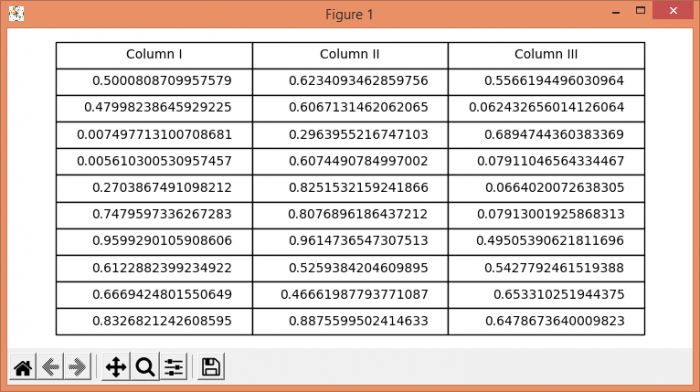# How to change the scale of an existing table in Matplotlib?

MatplotlibServer Side ProgrammingProgramming

To change scale of a table, we can use the scale() method. Steps −

• Create a figure and a set of subplots, nrows=1 and ncols=1.

• Create a random data using numpy.

• Make columns value.

• Make the axis tight and off.

• Initialize a variable fontsize to change the fontsize.

• To set the fontsize of the table and to scale the table, we can use 1.5 and 1.5.

• To display the figure, use the show() method.

## Example

import numpy as np
from matplotlib import pyplot as plt
plt.rcParams["figure.figsize"] = [7.50, 3.50]
plt.rcParams["figure.autolayout"] = True
fig, axs = plt.subplots(1, 1)
data = np.random.random((10, 3))
columns = ("Column I", "Column II", "Column III")
axs.axis('tight')
axs.axis('off')
fontsize = 10
the_table = axs.table(cellText=data, colLabels=columns, loc='center')
the_table.set_fontsize(fontsize)
the_table.scale(1.5, 1.5)
plt.show()

## Output